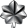## Sample code to FTP upload/download using SIM900 and Arduino

Saman
Lieutenant ColonelPosts: 828
Joined: Fri Jul 31, 2009 10:32 pm
Location: Mount Lavinia

### Sample code to FTP upload/download using SIM900 and Arduino

Code: Select all

``````/*
*  Description: This example shows how to upload and download files from a FTP
*  server. The example configures the module to use FTP funtions, uploads a file
*  to the FTP server and then downloads the content of the uploaded file and shows
*  it. This example only shows the AT commands (and the answers of the module)
*  used to use the FTP funtion and how work the FTP functions. For more
*  information about the AT commands, refer to the AT command manual.
*
*  Copyright (C) 2013 Libelium Comunicaciones Distribuidas S.L.
*  http://www.libelium.com
*
*  This program is free software: you can redistribute it and/or modify
*  it under the terms of the GNU General Public License as published by
*  the Free Software Foundation, either version 3 of the License, or
*  (at your option) any later version.
*
*  This program is distributed in the hope that it will be useful,
*  but WITHOUT ANY WARRANTY; without even the implied warranty of
*  MERCHANTABILITY or FITNESS FOR A PARTICULAR PURPOSE.  See the
*  GNU General Public License for more details.
*
*  You should have received a copy of the GNU General Public License
*  along with this program.  If not, see <http://www.gnu.org/licenses/>.
*
*  Version 0.2
*  Author: Alejandro Gallego
*/

int onModulePin = 2;
char aux_str;

char incoming_data;

char test_str[ ]= "0000000011111111222222223333333344444444555555556666666677777777000000001111111122222222333333334444";

int data_size, aux;

void setup(){

pinMode(onModulePin, OUTPUT);
Serial.begin(115200);

Serial.println("Starting...");
power_on();

delay(5000);

Serial.println("Connecting to the network...");

while( (sendATcommand("AT+CREG?", "+CREG: 0,1", 500)
|| sendATcommand("AT+CREG?", "+CREG: 0,5", 500)) == 0 );

configure_FTP();

Serial.print("Incoming data: ");
Serial.println(incoming_data);
}

void loop(){

}

void configure_FTP(){

sendATcommand("AT+SAPBR=3,1,\"Contype\",\"GPRS\"", "OK", 2000);
sendATcommand("AT+SAPBR=3,1,\"APN\",\"APN\"", "OK", 2000);
sendATcommand("AT+SAPBR=3,1,\"USER\",\"user_name\"", "OK", 2000);

while (sendATcommand("AT+SAPBR=1,1", "OK", 20000) != 1);
sendATcommand("AT+FTPCID=1", "OK", 2000);
sendATcommand("AT+FTPSERV=\"ftp.yourserver.com\"", "OK", 2000);
sendATcommand("AT+FTPPORT=21", "OK", 2000);
sendATcommand("AT+FTPUN=\"user_name\"", "OK", 2000);

}

sendATcommand("AT+FTPPUTNAME=\"file_name\"", "OK", 2000);
sendATcommand("AT+FTPPUTPATH=\"/path\"", "OK", 2000);
if (sendATcommand("AT+FTPPUT=1", "+FTPPUT:1,1,", 30000) == 1)
{
data_size = 0;
while(Serial.available()==0);
do{
data_size *= 10;
data_size += (aux-0x30);
while(Serial.available()==0);
}
while(aux != 0x0D);

if (data_size >= 100)
{
if (sendATcommand("AT+FTPPUT=2,100", "+FTPPUT:2,100", 30000) == 1)
{
Serial.println(sendATcommand(test_str, "+FTPPUT:1,1", 30000), DEC);
Serial.println(sendATcommand("AT+FTPPUT=2,0", "+FTPPUT:1,0", 30000), DEC);
}
else
{
sendATcommand("AT+FTPPUT=2,0", "OK", 30000);
}
}
else
{
sendATcommand("AT+FTPPUT=2,0", "OK", 30000);
}
}
else
{
Serial.println("Error openning the FTP session");
}
}

int x = 0;

sendATcommand("AT+FTPGETNAME=\"file_name\"", "OK", 2000);
sendATcommand("AT+FTPGETPATH=\"/path\"", "OK", 2000);
if (sendATcommand("AT+FTPGET=1 ", "+FTPGET:1,1", 30000) == 1)
{
do{
if (sendATcommand2("AT+FTPGET=2,50", "+FTPGET:2,", "+FTPGET:1,", 30000) == 1)
{
data_size = 0;
while(Serial.available()==0);
do{
data_size *= 10;
data_size += (aux-0x30);
while(Serial.available()==0);
}while(aux != 0x0D);

Serial.println(data_size);

if (data_size > 0)
{
while(Serial.available() < data_size);

for (int y = 0; y < data_size; y++)
{
x++;
}
incoming_data[x] = '\0';
}
else
{
}
}
else if (answer == 2)
{
Serial.println("Error from FTP");
}
else
{
Serial.println("Error getting the file");
data_size = 0;
}
}while (data_size > 0);
}
else
{
Serial.println("Error openning the FTP session");
}
}

void power_on(){

// checks if the module is started
answer = sendATcommand("AT", "OK", 2000);
if (answer == 0)
{
digitalWrite(onModulePin,HIGH);
delay(3000);
digitalWrite(onModulePin,LOW);

while(answer == 0){     // Send AT every two seconds and wait for the answer
answer = sendATcommand("AT", "OK", 2000);
}
}
}

int8_t sendATcommand(char* ATcommand, char* expected_answer, unsigned int timeout){

char response;
unsigned long previous;

memset(response, '\0', 100);    // Initialize the string

delay(100);

while( Serial.available() > 0) Serial.read();    // Clean the input buffer

Serial.println(ATcommand);    // Send the AT command

x = 0;
previous = millis();

// this loop waits for the answer
do{
if(Serial.available() != 0){
// if there are data in the UART input buffer, reads it and checks for the asnwer
//Serial.print(response[x]);
x++;
// check if the desired answer  is in the response of the module
if (strstr(response, expected_answer) != NULL)
{
}
}
}
// Waits for the asnwer with time out
while((answer == 0) && ((millis() - previous) < timeout));

}

int8_t sendATcommand2(char* ATcommand, char* expected_answer1,
char* expected_answer2, unsigned int timeout){

char response;
unsigned long previous;

memset(response, '\0', 100);    // Initialize the string

delay(100);

while( Serial.available() > 0) Serial.read();    // Clean the input buffer

Serial.println(ATcommand);    // Send the AT command

x = 0;
previous = millis();

// this loop waits for the answer
do{
// if there are data in the UART input buffer, reads it and checks for the asnwer
if(Serial.available() != 0){
x++;
// check if the desired answer 1 is in the response of the module
if (strstr(response, expected_answer1) != NULL)
{
}
// check if the desired answer 2 is in the response of the module
if (strstr(response, expected_answer2) != NULL)
{
}
}
// Waits for the asnwer with time out
}while((answer == 0) && ((millis() - previous) < timeout));